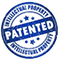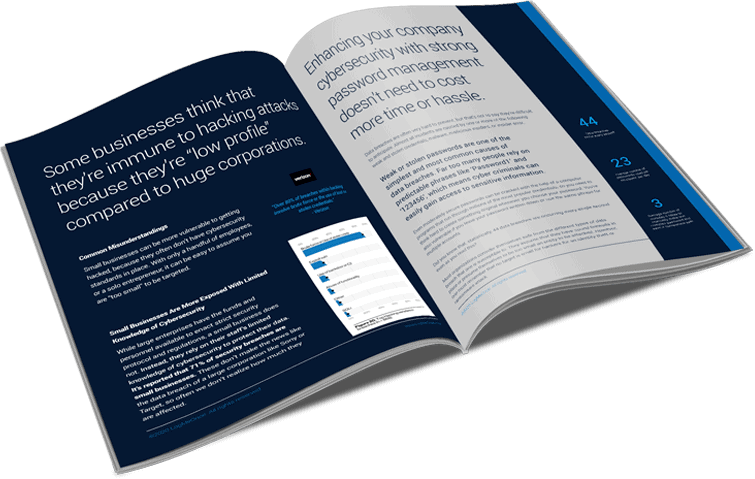``` (function(m,a,i,l,e,r){ m['MailerLiteObject']=e;function f(){ var c={ a:arguments,q:[]};var r=this.push(c);return "number"!=typeof r?r:f.bind(c.q);} f.q=f.q||[];m[e]=m[e]||f.bind(f.q);m[e].q=m[e].q||f.q;r=a.createElement(i); var _=a.getElementsByTagName(i);r.async=1;r.src=l+'?v'+(~~(new Date().getTime()/1000000)); _.parentNode.insertBefore(r,_);})(window, document, 'script', 'https://static.mailerlite.com/js/universal.js', 'ml'); var ml_account = ml('accounts', '1795852', 'w6k4n3s9b9', 'load'); ``` ``` var ml_webform_2576408 = ml_account('webforms', '2576408', 's7e1c5', 'load'); ml_webform_2576408('animation', 'fadeIn'); ```